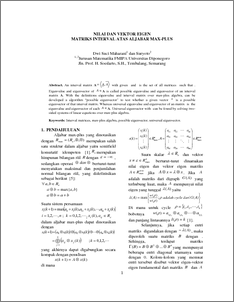# NILAI DAN VEKTOR EIGEN MATRIKS INTERVAL ATAS ALJABAR MAX-PLUS

Maharani, Dwi Suci and Suryoto, Suryoto (2009) NILAI DAN VEKTOR EIGEN MATRIKS INTERVAL ATAS ALJABAR MAX-PLUS. jURNAL MATEMATIKA DAN KOMPUTER . ISSN 1410-8518Preview
PDF
122Kb

## Abstract

An interval matrix A with given and is the set of all matrices such that . Eigenvalue and eigenvector of A is called possible eigenvalue and eigenvector of an interval matrix A. With the definitions eigenvalue and interval matrix over max-plus algebra, can be developed a algorithm “possible eigenvector” to test whether a given vector is a possible eigenvector of that interval matrix. Whereas universal eigenvalue and eigenvector of an matrix is the eigenvalue and eigenvector of each A. Universal eigenvector with can be found by solving two-sided systems of linear equations over max-plus algebra.

Item Type: Article Interval matrices, max-plus algebra, possible eigenvector, universal eigenvector. Q Science > QA Mathematics Faculty of Science and Mathematics > Department of Mathematics 1930 INVALID USER 30 Nov 2009 14:24 03 Dec 2009 16:30

Repository Staff Only: item control page